Water Resources of the United States

The following documentation was taken from:

U.S. Geological Survey Water-Resources Investigations Report 94-4002: Nationwide summary of U.S. Geological Survey regional regression equations for estimating magnitude and frequency of floods for ungaged sites, 1993

# INDIANA

## STATEWIDE RURAL

### Summary

Indiana is divided into seven hydrologic areas (fig. 1). The regression equations developed for these areas are for estimating peak discharges (QT) having recurrence intervals T that range from 2 to 100 years. The explanatory basin variables used in the equations are contributing drainage area (DA), in square miles; storage (STOR), which is the percentage of the contributing drainage area covered by lakes, ponds, and wetlands; mean annual precipitation (PREC), in inches; runoff coefficient (RC), which relates storm runoff to soil permeability; main-channel slope (SL), in feet per mile; precipitation (I24,2), in inches, the 2-year 24-hour precipitation; and main-channel length (L), in miles. The constants of 1, -30, and -2.5 are added to STOR, PREC, and I24,2 in the computer application of the regression equations. The user should enter the actual values of STOR, PREC, and I24,2. The variables DA, STOR, SL, and L can be measured from topographic maps. Variable PREC can be determined from figure 2; I24,2 can be determined from figure 3; and RC can be determined from figure 4. The regression equations were developed from peak-discharge records for 242 stations in Indiana, Ohio, and Illinois. The equations should be used only for unregulated, nonurbanized streams. Standard errors of estimate of the regression equations range from 24 to 45 percent. The report by Glatfelter (1984) includes flood-frequency data based on observed peaks for 270 gaged locations.

### Procedure

Topographic maps, the hydrologic area map (fig. 1), the mean annual precipitation map (fig. 2), the 2-year 24-hour precipitation map (fig. 3), the map showing major soil groups and runoff coefficients (fig. 4), and the following equations are used to estimate the needed peak discharges QT, in cubic feet per second, having selected recurrence intervals T.

### Area 5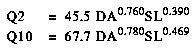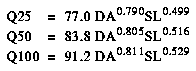### Area 6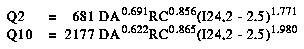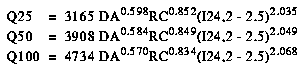### Area 7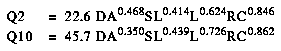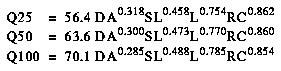### Reference

Glatfelter, D.R., 1984, Techniques for estimating magnitude and frequency of floods on streams in Indiana: U.S. Geological Survey Water-Resources Investigations Report 84-4134, 110 p.

Figure 1. Flood-frequency region map for Indiana. (PostScript file of Figure 1.)

Figure 2. Mean annual precipitation in Indiana. (PostScript file of Figure 2.)

Figure 3. The 2-year 24-hour precipitation in Indiana. (PostScript file of Figure 3.)

Figure 4. Hydrologic soil-groups map for Indiana. (PostScript file of Figure 4.)U.S. Department of the Interior | U.S. Geological Survey
URL: https://water.usgs.gov/software/NFF/manual/in/
[an error occurred while processing this directive] Page Contact Information: pacampbe@usgs.gov